﻿ Concrete properties machine learning example

# Model the compressive strength of high performance concretes using machine learning

Compressive strength is one of the most important properties of concrete.

It is measured by breaking cylindrical concrete specimens in a compression-testing machine.

This example aims to design concrete mixtures with specified properties and reduced costs.

To do that, we build a compressive strength's predictive model from a set of tests performed in the laboratory for 425 specimens.### Contents:

This example is solved with Neural Designer. To follow it step by step, you can use the free trial .

## 1. Application type

The variable to be predicted is continuous (compressive strength). Therefore, this is an approximation project

The primary goal here is to model the compressive strength as a function of the concrete components.

## 2. Data set

The first step is to prepare the data set , which is the source of information for the approximation problem. It is composed of:

• Data file.
• Variables information.
• Instances information.

The data file concrete_properties.csv contains 8 columns and 425 rows.

The next listing shows the variables in the data set and their use:

• cement, in kg/m3.
• blast_furnace_slag, in kg/m3.
• fly_ash, in kg/m3.
• water, in kg/m3.
• superplasticizer, in kg/m3.
• coarse_aggregate, in kg/m3.
• fine_aggregate, in kg/m3.
• compressive_strength, in MPa.

The last variable, compressive_strength, will be set as the target we aim to model. Its value gives valuable insight into the quality of a specific concrete mixture.

The rest of the variables, which depict the densities (mass per unit of mixture volume) of the different materials put together to form concrete, will be used as inputs for the neural network.

The instances are divided into training, selection, and testing subsets. They represent 60%, 20%, and 20% of the original instances, respectively, and are split at random.

Once we have set all the data set information, we are ready to perform some analytics to check the quality of the data.

For instance, we can calculate the data distribution. The next figure depicts the histogram for the target variable.As we can see, the compressive strength has a normal distribution.

The next figure depicts inputs-targets correlations . This might help us see the influence of the different inputs on the concrete's compressive strength.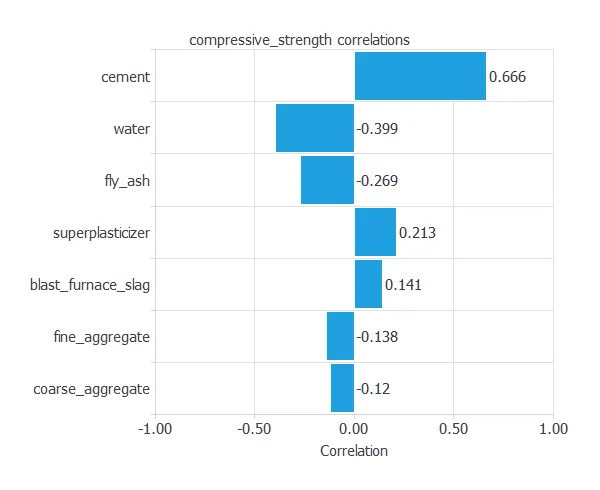The above chart shows that cement has the greatest impact on compressive strength.

We can also plot a scatter chart with the compressive strength versus the cement amount.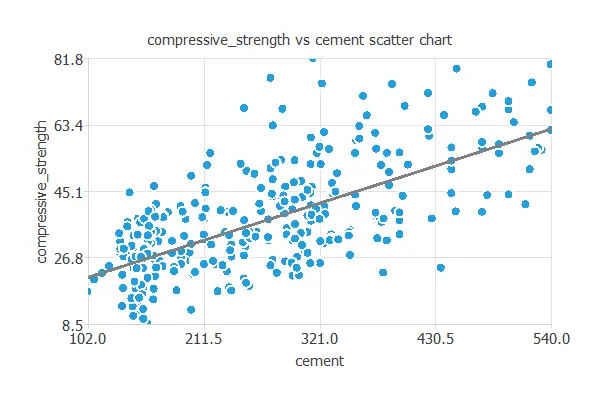In general, the more cement, the more compressive strength. However, the compressive strength depends on all the inputs simultaneously.

## 3. Neural network

The second step is to set the neural network stuff. For approximation project types, a neural network is usually composed by:

• Scaling layer.
• Perceptron layers.
• Unscaling layer.

The scaling layer contains the statistics on the inputs calculated from the data file and the method for scaling the input variables. Here the minimum and maximum scaling method has been set. Nevertheless, the mean-standard deviation method would produce very similar results.

Here two perceptron layers are added to the neural network. This number of layers is enough for most applications. The first layer has five inputs and three neurons. The second layer has three inputs and one neuron. Hyperbolic tangent and linear functions have been set as the activation functions for the first and second layers. These are the default values we will be using as a first guess.

The unscaling layer transforms the normalized values from the neural network into the original outputs. Here the minimum and maximum unscaling method will also be used.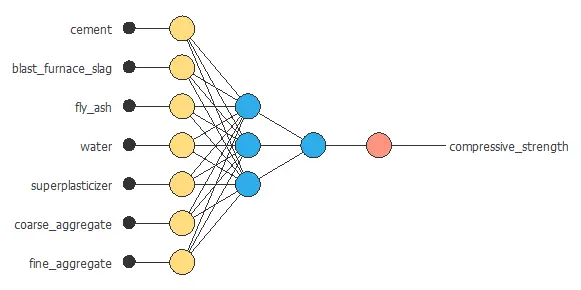The figure above shows the resulting network architecture.

## 4. Training strategy

The fourth step is to select an appropriate training strategy. It is composed of two parameters:

• A loss index.
• An optimization algorithm.

The normalized squared error divides the squared error between the outputs from the neural network and the targets in the data set by a normalization coefficient. If the normalized squared error has a value of 1, then the neural network predicts the data 'in the mean', while a value of zero means the perfect prediction of the data. This error term does not have any parameters to set.

The L2 regularization is applied to control the neural network's complexity by reducing the value of the parameters. A weak regularization weight is applied here.

The learning problem can be stated as finding a neural network that minimizes the loss index. That is, a neural network that fits the data set (error term) and does not oscillate (regularization term).

The next step in solving this problem is to assign the optimization algorithm . We use the quasi-Newton method here.

The neural network is trained to obtain the best possible performance. The next table shows the training history.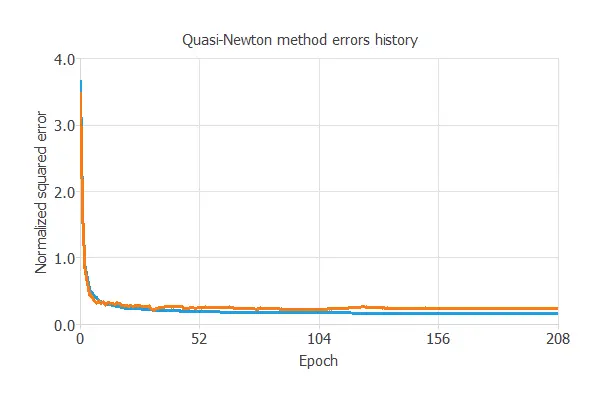The final training and selection errors are training error = 0.153 NSE and selection error = 0.24 NSE , respectively. The next section will improve the generalization performance by reducing the selection error.

## 5. Model selection

The best generalization is achieved using a model with the most appropriate complexity to produce an adequate data fit. Order selection is responsible for finding the optimal number of perceptrons. The algorithm selected for this purpose is the incremental order method.

The next image shows the result after the process. The blue line symbolizes the training error, and the orange represents the selection error.As shown in the picture, the method starts with a small number of neurons (order) and increases the complexity at each iteration. The algorithm selects the order with the minimum selection loss. The selection error increases for greater values than this order due to overfitting since it would be a complex model.

After the Order selection , we have achieved a selection error of 0.211839 NSE.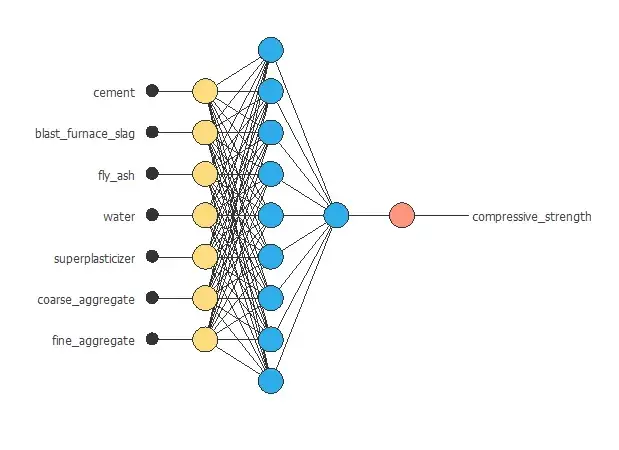The figure above represents the final network architecture .

### 6. Testing analysis

A standard method for testing the approximation model's prediction capabilities is to compare the outputs from the neural network against an independent set of data.

The next plot shows the predicted compressive strength values versus the actual ones.As we can see, both values are very similar to the entire range of data. The correlation coefficient is R2 = 0.861, which indicates that the model has a reliable prediction capability.

It is also convenient to explore the errors made by the neural network on single testing instances. Some outliers are removed to achieve the best possible performance in this example. The mean error is 5.53%, with a standard deviation of 3.69%, which is a good value for this application.

## 7. Model deployment

Once we know that the neural network can accurately predict the compressive strength, we can move to the model deployment phase to design concretes with desired properties.

For that purpose, we can use Response Optimization . The objective of the response optimization algorithm is to exploit the mathematical model to look for optimal operating conditions.
Indeed, the predictive model allows us to simulate different operating scenarios and adjust the control variables to improve efficiency.

An example is to minimize the cement amount while maintaining the compressive strength over the desired value.

The next table resumes the conditions for this problem.

Variable name Condition
Cement Minimize
Blast furnace slag None
Fly ash None
Water None
superplasticizer None
Coarse aggregate None
Fine aggregate None
Compressive strength Greater than or equal to 45

The next list shows the optimum values for previous conditions.

• cement: 104.152 kg/m3.
• blast_furnace_slag: 191.896 kg/m3.
• fly_ash: 99.7447 kg/m3.
• water: 217.442 kg/m3.
• superplasticizer: 17.6654 kg/m3.
• coarse_aggregate: 1068.33 kg/m3.
• fine_aggregate: 939.43 kg/m3.
• compressive_strength: 51.5842 MPa.

It is advantageous to see how the outputs vary as a single input function when all the others are fixed. Directional outputs plot the neural network outputs through some reference points.

The next list shows the reference point for the plots.

• cement: 265 kg/m3.
• blast_furnace_slag: 86 kg/m3.
• fly_ash: 62 kg/m3.
• water: 183 kg/m3.
• superplasticizer: 7 kg/m3.
• coarse_aggregate: 956 kg/m3.
• fine_aggregate: 764 kg/m3.

The following plot shows how the compressive strength varies with the cement amount for that reference point.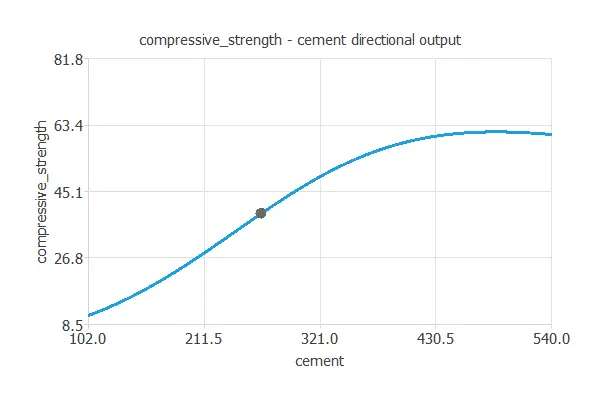The next listing is the mathematical expression represented by the predictive model.

```scaled_cement = (cement-265.444)/104.67;
scaled_blast_furnace_slag = (blast_furnace_slag-86.2852)/87.8265;
scaled_fly_ash = (fly_ash-62.7953)/66.2277;
scaled_water = (water-183.06)/19.3286;
scaled_superplasticizer = (superplasticizer-6.99576)/5.39228;
scaled_coarse_aggregate = (coarse_aggregate-956.059)/83.8016;
scaled_fine_aggregate = (fine_aggregate-764.377)/73.1205;
y_1_1 = tanh (0.00514643+ (scaled_cement*-0.252051)+ (scaled_blast_furnace_slag*0.219995)+ (scaled_fly_ash*0.21738)+ (scaled_water*0.409424)+ (scaled_superplasticizer*-0.582195)+ (scaled_coarse_aggregate*0.228467)+ (scaled_fine_aggregate*-0.0963871));
y_1_2 = tanh (-0.66057+ (scaled_cement*-0.381739)+ (scaled_blast_furnace_slag*-0.303248)+ (scaled_fly_ash*-0.153626)+ (scaled_water*-0.29632)+ (scaled_superplasticizer*-0.0808802)+ (scaled_coarse_aggregate*-0.305395)+ (scaled_fine_aggregate*-0.202458));
y_1_3 = tanh (-0.0348251+ (scaled_cement*0.105028)+ (scaled_blast_furnace_slag*-0.518262)+ (scaled_fly_ash*-0.54546)+ (scaled_water*0.0903926)+ (scaled_superplasticizer*1.03298)+ (scaled_coarse_aggregate*0.0226592)+ (scaled_fine_aggregate*0.247202));
scaled_compressive_strength =  (-1.25097+ (y_1_1*-1.11323)+ (y_1_2*-1.90226)+ (y_1_3*-0.770388));
compressive_strength = (0.5*(scaled_compressive_strength+1.0)*(81.75-8.54)+8.54);
```

The above formula can be exported to the software tool required by the customer.

The purpose of improving the quality of concrete was to help construction companies to obtain the best product suited to their needs at a minimum cost. We have used a neural network to model 425 specimens of concrete, to predict the compressive strength as a function of the constituent materials and their proportions.

## 8. Video tutorial

You can watch the step-by-step tutorial video below to help you complete this Machine Learning example for free using the powerful machine learning software, Neural Designer.

### References

• I-Cheng Yeh, "Modeling of strength of high performance concrete using artificial neural networks", Cement and Concrete Research, Vol. 28, No. 12, pp. 1797-1808 (1998).